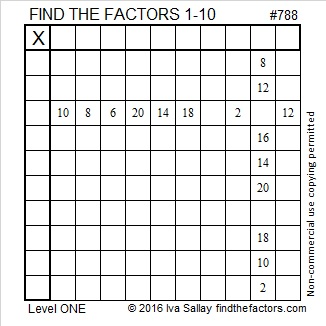# 788 and Level 1

Since 88, its last two digits, are divisible by 4, we know that 788 and every other whole number ending in 88 is divisible by 4.

I learned the following fascinating fact about these six numbers starting with 788 from Stetson.edu:788 is also palindrome 404 in BASE 14. Note that 4(196) + 0(14) + 4(1) = 788.

788 is the hypotenuse of Pythagorean triple 112-780-788 which is 28-195-197 times 4.Print the puzzles or type the solution on this excel file: 10-factors-788-794

• 788 is a composite number.
• Prime factorization: 788 = 2 x 2 x 197, which can be written 788 = (2^2) x 197
• The exponents in the prime factorization are 2 and 1. Adding one to each and multiplying we get (2 + 1)(1 + 1) = 3 x 2  = 6. Therefore 788 has exactly 6 factors.
• Factors of 788: 1, 2, 4, 197, 394, 788
• Factor pairs: 788 = 1 x 788, 2 x 394, or 4 x 197
• Taking the factor pair with the largest square number factor, we get √788 = (√4)(√197) = 2√197 ≈ 28.071338.